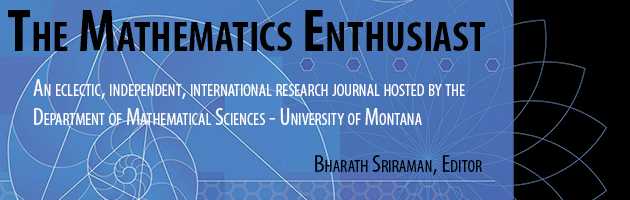•
•#### Article Title

Exploring Perimeter and Area with 4th Graders

1

1

#### Abstract

I am going to teach basic introductory geometry skills to 4th graders using Geometry’s Sketchpad. At this age, children are only beginning to learn about geometry in their math classes, and I would like for the students to understand these basic concepts using technology. This problem is so important for the students to learn early, and learn correctly. These skills will involve finding the area and perimeter of regular polygons, basic skills using Geometry’s Sketchpad, and activities that will apply these introductory concepts; directly correlating within the national geometry standards of mathematics. Children use geometry everyday, even when they don’t realize they are using them (actually we all do). But the most important concept for the children is to recognize shapes and structures. If they can identify the use of geometry, then it will automatically trigger the children to think; think in a mathematical state of mind. Thinking mathematically creates questions and also answers questions.

With Geometry’s Sketchpad, children can learn these skills much earlier because they can construct, observe, calculate and assess everything independently. Fortunately this contemporary technology is becoming more available in elementary classrooms. These concepts are most likely new to the students at the lower levels; therefore, teaching basic Geometry’s Sketchpad skills to 4th graders will be a great introduction to beginning geometry. According to the national geometry standards for fourth graders, children will be able to analyze characteristic and properties of two-dimensional geometric shapes and develop mathematical arguments about geometric relationships. In these activities, I will explain the basic concepts of perimeters and areas of polygons with and without Geometry’s Sketchpad. I will then give specific instructions on how to use the Sketchpad, next I will transition into three fun activities where the students can use what they’ve learned and understand how these skills can be associated with their own lives. These activities will include the national standards by having the children specify locations and describe spatial relationships using coordinate on Geometer’s Sketchpad and other representational systems such as the geoboards. This will not only give students a fundamental introduction to geometry, but will provide them with knowledge for future application. Most importantly, students will become aware how mathematics and specifically geometry can be used in their everyday lives. Again incorporating these national standards by using visualization, spatial reasoning, geometric modeling to solve problems and apply the problems to analyze mathematical situations.

14

19

#### Digital Object Identifier (DOI)

10.54870/1551-3440.1003

COinS